# 7 款 Python 数据图表工具的比较

Python 的科学栈相当成熟，各种应用场景都有相关的模块，包括机器学习和数据分析。数据可视化是发现数据和展示结果的重要一环，只不过过去以来，相对于 R 这样的工具，发展还是落后一些。

### 探索数据集

# Import the pandas library.
import pandas
# Read in the airports data.
airports.columns = ["id", "name", "city", "country", "code", "icao", "latitude", "longitude", "altitude", "offset", "dst", "timezone"]
# Read in the airlines data.
airlines.columns = ["id", "name", "alias", "iata", "icao", "callsign", "country", "active"]
# Read in the routes data.
routes.columns = ["airline", "airline_id", "source", "source_id", "dest", "dest_id", "codeshare", "stops", "equipment"]

airports.head()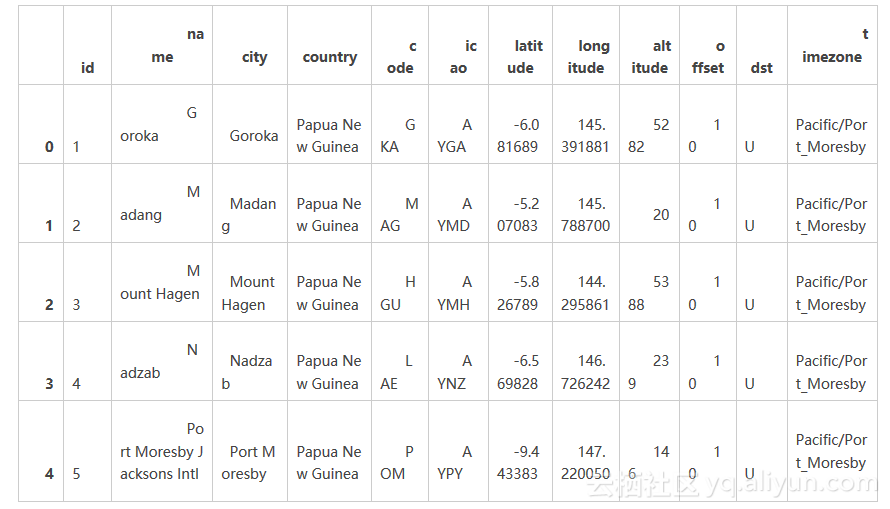airlines.head()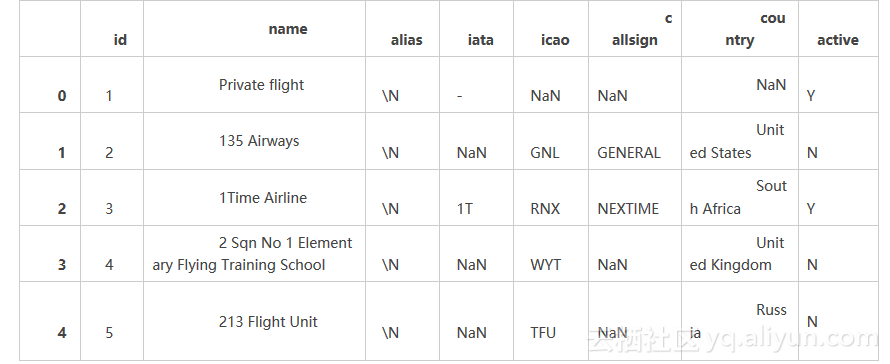routes.head()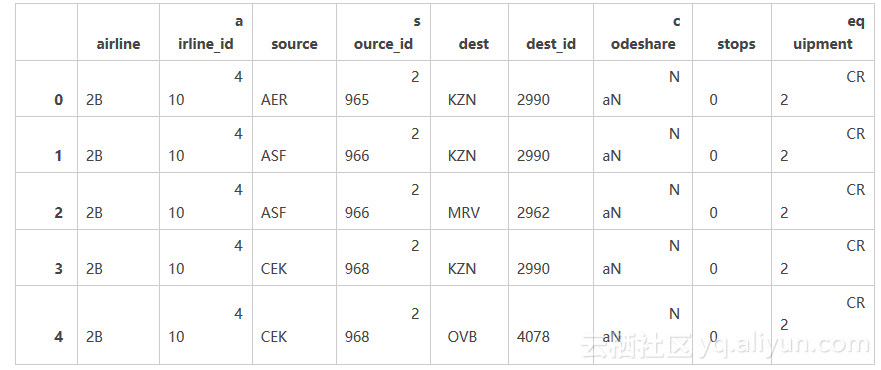routes = routes[routes["airline_id"] != "\\N"]

### 制作柱状图

import math
def haversine(lon1, lat1, lon2, lat2):
# Convert coordinates to floats.
lon1, lat1, lon2, lat2 = [float(lon1), float(lat1), float(lon2), float(lat2)]
# Convert to radians from degrees.
lon1, lat1, lon2, lat2 = map(math.radians, [lon1, lat1, lon2, lat2])
# Compute distance.
dlon = lon2 - lon1
dlat = lat2 - lat1
a = math.sin(dlat/2)**2 + math.cos(lat1) * math.cos(lat2) * math.sin(dlon/2)**2
c = 2 * math.asin(math.sqrt(a))
km = 6367 * c
return km

def calc_dist(row):
dist = 0
try:
# Match source and destination to get coordinates.
source = airports[airports["id"] == row["source_id"]].iloc
dest = airports[airports["id"] == row["dest_id"]].iloc
# Use coordinates to compute distance.
dist = haversine(dest["longitude"], dest["latitude"], source["longitude"], source["latitude"])
except (ValueError, IndexError):
pass
return dist

route_lengths = routes.apply(calc_dist, axis=1)

import matplotlib.pyplot as plt
%matplotlib inline

plt.hist(route_lengths, bins=20)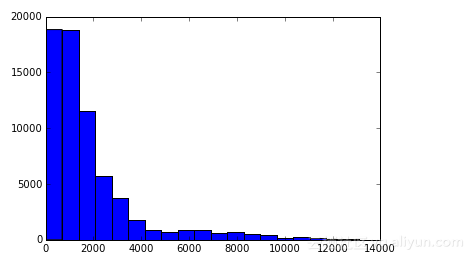### 使用 seaborn

import seaborn
seaborn.distplot(route_lengths, bins=20)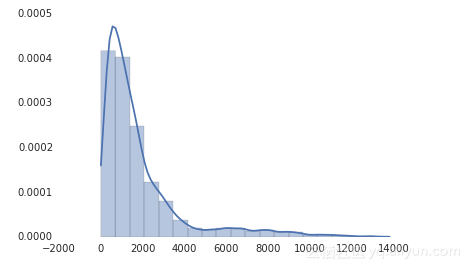### 条形图

import numpy
# Put relevant columns into a dataframe.
route_length_df = pandas.DataFrame({"length": route_lengths, "id": routes["airline_id"]})
# Compute the mean route length per airline.
airline_route_lengths = route_length_df.groupby("id").aggregate(numpy.mean)
# Sort by length so we can make a better chart.
airline_route_lengths = airline_route_lengths.sort("length", ascending=False)

plt.bar(range(airline_route_lengths.shape), airline_route_lengths["length"])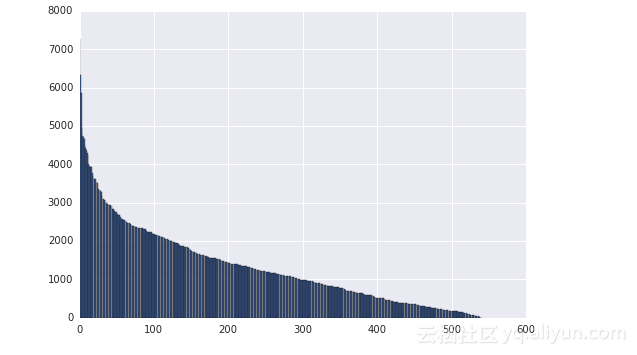Matplotlib的plt.bar方法根据每个数据模型的航空公司平均航线长度（airline_route_lengths["length"]）来做图。

def lookup_name(row):
try:
# Match the row id to the id in the airlines dataframe so we can get the name.
name = airlines["name"][airlines["id"] == row["id"]].iloc
except (ValueError, IndexError):
name = ""
return name
# Add the index (the airline ids) as a column.
airline_route_lengths["id"] = airline_route_lengths.index.copy()
# Find all the airline names.
airline_route_lengths["name"] = airline_route_lengths.apply(lookup_name, axis=1)
# Remove duplicate values in the index.
airline_route_lengths.index = range(airline_route_lengths.shape)

import numpy as np
from bokeh.io import output_notebook
from bokeh.charts import Bar, show
output_notebook()
p = Bar(airline_route_lengths, 'name', values='length', title="Average airline route lengths")
show(p)

### 水平条形图

Pygal 是一个能快速制作出有吸引力表格的数据分析库。我们可以用它来按长度分解路由。首先把我们的路由分成短、中、长三个距离，并在 route_lengths 里计算出它们各占的百分比。

long_routes = len([k for k in route_lengths if k > 10000]) / len(route_lengths)
medium_routes = len([k for k in route_lengths if k < 10000 and k > 2000]) / len(route_lengths)
short_routes = len([k for k in route_lengths if k < 2000]) / len(route_lengths)

import pygal
from IPython.display import SVG
chart = pygal.HorizontalBar()
chart.title = 'Long, medium, and short routes'
chart.render_to_file('routes.svg')
SVG(filename='routes.svg')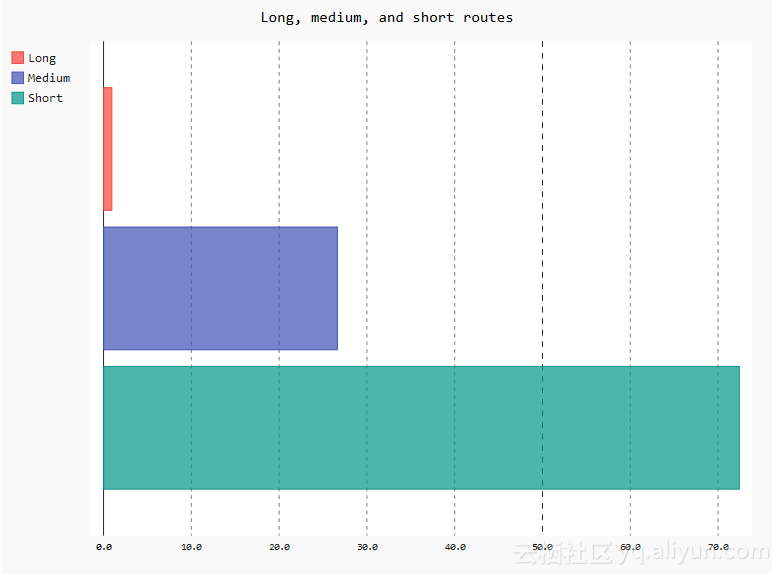### 散点图

name_lengths = airlines["name"].apply(lambda x: len(str(x)))
plt.scatter(airlines["id"].astype(int), name_lengths)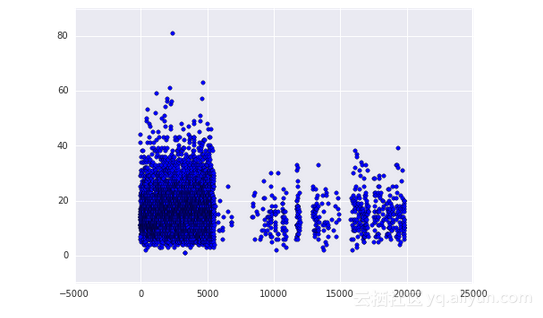data = pandas.DataFrame({"lengths": name_lengths, "ids": airlines["id"].astype(int)})
seaborn.jointplot(x="ids", y="lengths", data=data)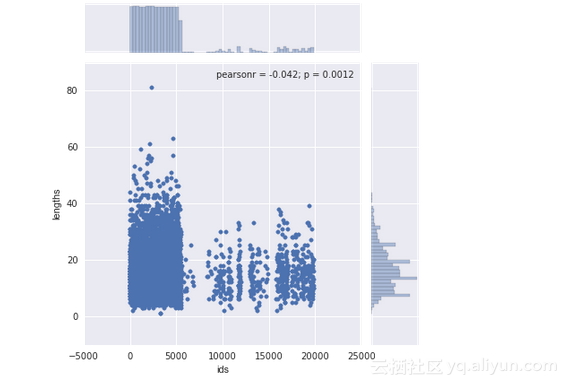### 静态 maps

# Import the basemap package
from mpl_toolkits.basemap import Basemap
# Create a map on which to draw.  We're using a mercator projection, and showing the whole world.
m = Basemap(projection='merc',llcrnrlat=-80,urcrnrlat=80,llcrnrlon=-180,urcrnrlon=180,lat_ts=20,resolution='c')
# Draw coastlines, and the edges of the map.
m.drawcoastlines()
m.drawmapboundary()
# Convert latitude and longitude to x and y coordinates
x, y = m(list(airports["longitude"].astype(float)), list(airports["latitude"].astype(float)))
# Use matplotlib to draw the points onto the map.
m.scatter(x,y,1,marker='o',color='red')
# Show the plot.
plt.show()

import folium
# Get a basic world map.
airports_map = folium.Map(location=[30, 0], zoom_start=2)
# Draw markers on the map.
for name, row in airports.iterrows():
# For some reason, this one airport causes issues with the map.
if row["name"] != "South Pole Station":
airports_map.circle_marker(location=[row["latitude"], row["longitude"]], popup=row["name"])
# Create and show the map.
airports_map.create_map('airports.html')
airports_map

Folium 使用 leaflet.js 来制作全交互式地图。你可以点击每一个机场在弹出框中看名字。在上边显示一个截屏，但是实际的地图更令人印象深刻。Folium 也允许非常广阔的修改选项来做更好的标注，或者添加更多的东西到地图上。

### 画弧线

# Make a base map with a mercator projection.  Draw the coastlines.
m = Basemap(projection='merc',llcrnrlat=-80,urcrnrlat=80,llcrnrlon=-180,urcrnrlon=180,lat_ts=20,resolution='c')
m.drawcoastlines()
# Iterate through the first 3000 rows.
for name, row in routes[:3000].iterrows():
try:
# Get the source and dest airports.
source = airports[airports["id"] == row["source_id"]].iloc
dest = airports[airports["id"] == row["dest_id"]].iloc
# Don't draw overly long routes.
if abs(float(source["longitude"]) - float(dest["longitude"])) < 90:
# Draw a great circle between source and dest airports.
m.drawgreatcircle(float(source["longitude"]), float(source["latitude"]), float(dest["longitude"]), float(dest["latitude"]),linewidth=1,color='b')
except (ValueError, IndexError):
pass

# Show the map.
plt.show()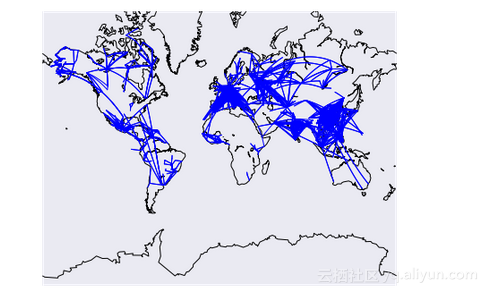### 画网络图

# Initialize the weights dictionary.
weights = {}
# Keep track of keys that have been added once -- we only want edges with a weight of more than 1 to keep our network size manageable.
# Iterate through each route.
for name, row in routes.iterrows():
# Extract the source and dest airport ids.
source = row["source_id"]
dest = row["dest_id"]

# Create a key for the weights dictionary.
# This corresponds to one edge, and has the start and end of the route.
key = "{0}_{1}".format(source, dest)
# If the key is already in weights, increment the weight.
if key in weights:
weights[key] += 1
# If the key is in added keys, initialize the key in the weights dictionary, with a weight of 2.
weights[key] = 2
# If the key isn't in added_keys yet, append it.
# This ensures that we aren't adding edges with a weight of 1.
else:
added_keys.append(key)

# Import networkx and initialize the graph.
import networkx as nx
graph = nx.Graph()
# Keep track of added nodes in this set so we don't add twice.
nodes = set()
# Iterate through each edge.
for k, weight in weights.items():
try:
# Split the source and dest ids and convert to integers.
source, dest = k.split("_")
source, dest = [int(source), int(dest)]
# Add the source if it isn't in the nodes.
if source not in nodes:
# Add the dest if it isn't in the nodes.
if dest not in nodes:
# Add both source and dest to the nodes set.
# Sets don't allow duplicates.

# Add the edge to the graph.
except (ValueError, IndexError):
pass
pos=nx.spring_layout(graph)
# Draw the nodes and edges.
nx.draw_networkx_nodes(graph,pos, node_color='red', node_size=10, alpha=0.8)
nx.draw_networkx_edges(graph,pos,width=1.0,alpha=1)
# Show the plot.
plt.show()

### 总结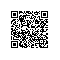使用钉钉扫一扫加入圈子
+ 订阅